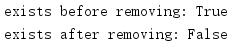# 1. pathlib文件系统路径作为对象

pathlib模块提供了一个面向对象API来解析、建立、测试和处理文件名和路径，而不是使用底层字符串操作。

## 1.1 路径表达

pathlib包含一些类来管理使用POSIX标准或Microsoft Windows语法格式化的文件系统路径。这个模块包含一些“纯”类，会处理字符串但不与实际的文件系统交互，另外还包含一些“具体”类，它们扩展了API，以包含可以反映或修改本地文件系统上数据的操作。

## 1.2 建立路径

import pathlib

usr = pathlib.PurePosixPath('/usr')
print(usr)

usr_local = usr / 'local'
print(usr_local)

usr_share = usr / pathlib.PurePosixPath('share')
print(usr_share)

root = usr / '..'
print(root)

etc = root / '/etc/'
print(etc)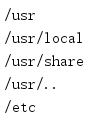import pathlib

usr_local = pathlib.Path('/usr/local')
share = usr_local / '..' / 'share'
print(share.resolve())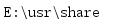import pathlib

root = pathlib.PurePosixPath('/')
subdirs = ['usr', 'local']
usr_local = root.joinpath(*subdirs)
print(usr_local)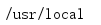import pathlib

ind = pathlib.PurePosixPath('source/pathlib/index.rst')
print(ind)

py = ind.with_name('pathlib_from_existing.py')
print(py)

pyc = py.with_suffix('.pyc')
print(pyc)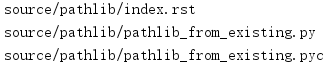## 1.3 解析路径

import pathlib

p = pathlib.PurePosixPath('/usr/local')
print(p.parts)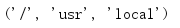import pathlib

p = pathlib.PurePosixPath('/usr/local/lib')

print('parent: {}'.format(p.parent))

print('\nhierarchy:')
for up in p.parents:
print(up)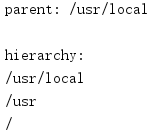import pathlib

p = pathlib.PurePosixPath('./source/pathlib/pathlib_name.py')
print('path  : {}'.format(p))
print('name  : {}'.format(p.name))
print('suffix: {}'.format(p.suffix))
print('stem  : {}'.format(p.stem))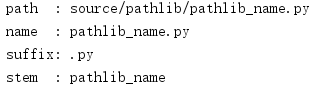## 1.4 创建具体路径

import pathlib

home = pathlib.Path.home()
print('home: ', home)

cwd = pathlib.Path.cwd()
print('cwd : ', cwd)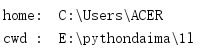## 1.5 目录内容

import pathlib

p = pathlib.Path('.')

for f in p.iterdir():
print(f)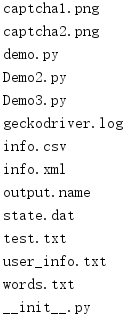import pathlib

p = pathlib.Path('..')

for f in p.glob('*.py'):
print(f)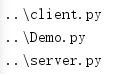glob处理器支持使用模式前缀**或者通过调用rglob()而不是glob()来完成递归扫描。

import pathlib

p = pathlib.Path('..')

for f in p.rglob('*.py'):
print(f)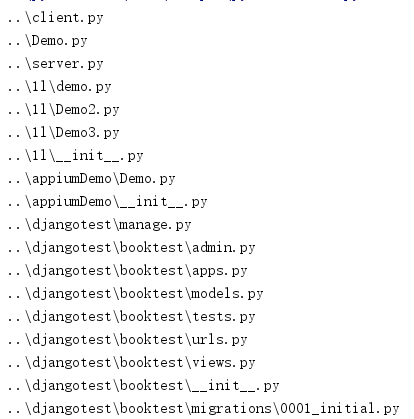## 1.6 读写文件

import pathlib

f = pathlib.Path('example.txt')

f.write_bytes('This is the content'.encode('utf-8'))

with f.open('r', encoding='utf-8') as handle:

print('read_text(): {!r}'.format(f.read_text('utf-8')))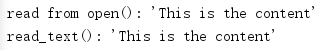## 1.7 管理目录和符号链接

import pathlib

p = pathlib.Path('example_dir')

print('Creating {}'.format(p))
p.mkdir()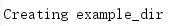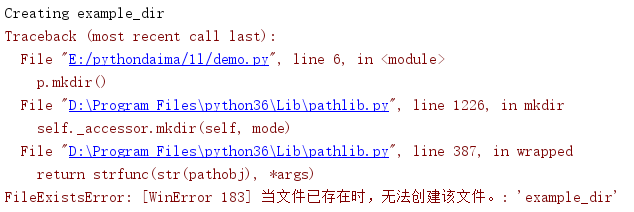import pathlib

print(p)
print(p.resolve().name)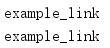## 1.8 文件类型

Path实例包含一些方法来检查路径指示的文件的类型。下面这个例子测试它们。

import pathlib

p = pathlib.Path('demo.py')

hfmt = '{:18s}' + ('  {:>5}' * 6)
print(hfmt.format('Name', 'File', 'Dir', 'Link', 'FIFO', 'Block',
'Character'))
print()
fmt = '{:20s}  ' + ('{!r:>5}  ' * 6)
print(fmt.format(
str(p),
p.is_file(),
p.is_dir(),
p.is_fifo(),
p.is_block_device(),
p.is_char_device(),
))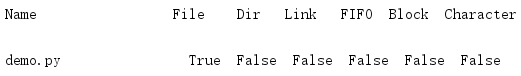## 1.9 文件属性

import pathlib
import sys
import time

if len(sys.argv) == 1:
filename = __file__
else:
filename = sys.argv

p = pathlib.Path(filename)
stat_info = p.stat()

print('{}:'.format(filename))
print('  Size:', stat_info.st_size)
print('  Permissions:', oct(stat_info.st_mode))
print('  Owner:', stat_info.st_uid)
print('  Device:', stat_info.st_dev)
print('  Created      :', time.ctime(stat_info.st_ctime))
print('  Last accessed:', time.ctime(stat_info.st_atime))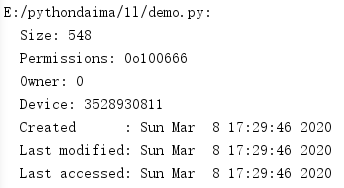## 1.10 删除

import pathlib

p = pathlib.Path('example_dir')

print('Removing {}'.format(p))
p.rmdir()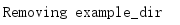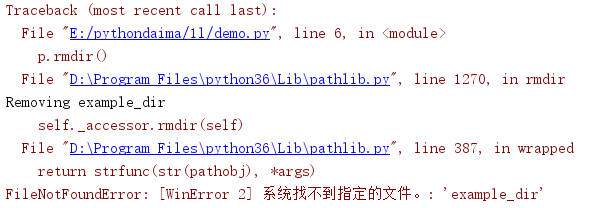import pathlib

p = pathlib.Path('touched')

p.touch()

print('exists before removing:', p.exists())

print('exists after removing:', p.exists())